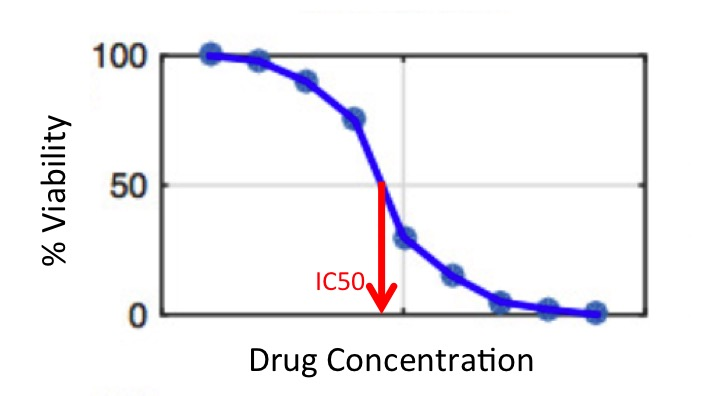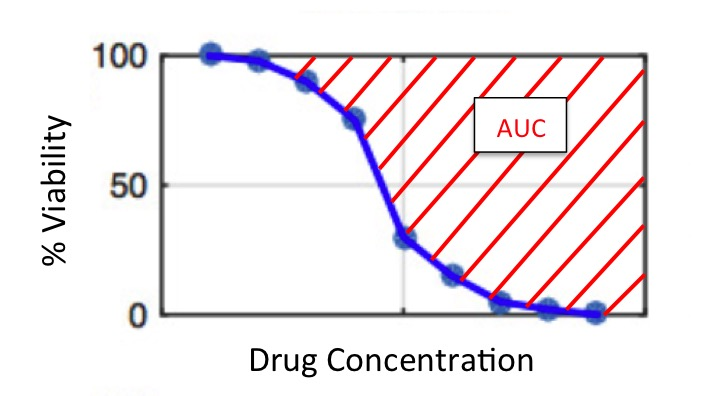## Introduction

Hopefully you have had the chance to explore some of the features of the CCLE and GDSC drug response datasets. If not, it might be a good idea to check out Tutorial 1a (“Exploring Pharmacological Data with the `rawPharmacData` Dataset”) to get a feel for the types of variables contained in the raw data. In contrast to the raw data which include the viability at each drug concentration for each cell line, the summarized dataset contains numerical summaries of each cell line’s response to each drug over all concentrations.

In this tutorial we’ll first learn more about summary measures of drug response, and then use scatterplots and correlation measures to assess the agreement of these summary measures in the two studies.

### Summary Measures

In the summarized dataset, the cell line viability over all drug concentrations has been summarized into a single number for each cell line and drug combination. This summary represents the overall effect of the drug on the cell line. There are many different ways this could be done. Our data includes two summary measures that were used in the original studies:

1. IC50 (Half Maximal Inhibitory Concentration): the estimated concentration of the drug that will result in half (50%) of the cells surviving.Are cell lines with higher IC50 values more or less susceptible? What about drugs with higher IC50 values - are they more or less toxic?

1. AUC (Area Under the Curve): despite the name, this is actually the area above the curve estimated by the drug concentration and viability data. Note that the estimation of this curve is not a simple task in itself - check out the tutorial on summarizing the relationship between two variables to learn more (Tutorial 2b).Are cell lines with higher values of AUC more or less resistant?

Are drugs with higher AUC more or less toxic?

## Setup Workspace

We start by loading the tidyverse family of packages and specifying a default plotting theme for our `ggplot` graphics.

``library(tidyverse)``
``````## Registered S3 methods overwritten by 'ggplot2':
##   method         from
##   [.quosures     rlang
##   c.quosures     rlang
##   print.quosures rlang``````
``## ── Attaching packages ────────────────────────────────── tidyverse 1.2.1 ──``
``````## ✔ ggplot2 3.1.1     ✔ purrr   0.3.2
## ✔ tibble  2.1.2     ✔ dplyr   0.8.1
## ✔ tidyr   0.8.3     ✔ stringr 1.4.0
## ✔ readr   1.3.1     ✔ forcats 0.4.0``````
``````## ── Conflicts ───────────────────────────────────── tidyverse_conflicts() ──
``theme_set(theme_bw())``

Let’s start by loading the `RDS` file containing the summarized pharmacological data (including the IC50 and AUC values for each drug and cell line combination, as described above).

``summarizedData <- readRDS(file.path("..", "data", "summarizedPharmacoData.rds"))``

As we did with the raw data, we’ll take a quick peek at this data before getting started.

``str(summarizedData)``
``````## 'data.frame':    2557 obs. of  6 variables:
##  \$ cellLine : chr  "22RV1" "5637" "639-V" "697" ...
##  \$ drug     : chr  "Nilotinib" "Nilotinib" "Nilotinib" "Nilotinib" ...
##  \$ ic50_CCLE: num  8 7.48 8 1.91 8 ...
##  \$ auc_CCLE : num  0 0.00726 0.07101 0.15734 0 ...
##  \$ ic50_GDSC: num  155.27 219.93 92.18 3.06 19.63 ...
##  \$ auc_GDSC : num  0.00394 0.00362 0.00762 0.06927 0.02876 ...``````

We can count the number of cell lines and drugs in the data.

``````## with base R
length(unique(summarizedData\$cellLine))``````
``##  288``
``length(unique(summarizedData\$drug))``
``##  15``
``````## with the tidyverse
summarizedData %>%
summarize(nCellLines = n_distinct(cellLine),
nDrugs     = n_distinct(drug))``````
``````##   nCellLines nDrugs
## 1        288     15``````

Notice that there are 2557 rows - each row here corresponds to a cell line-drug combination. Making up these combinations are 288 unique cell lines, and 15 drugs.

Was every cell line in the dataset tested with every drug?

## Comparing Studies using Plots

So we now have summary measures (IC50 and AUC) that indicate the responses of cell lines to drugs. However, each study measured these values separately. The goal of our analysis is to investigate how well these two studies agree with each other. In other words, do the drug response results in one study replicate in the other study?

### AZD0530

First, we’ll examine this question for one of the drugs in particular: AZD0530. To make our code easier to read, let’s create a separate object from for this subset of the data and call it `azdSummary`.

``azdSummary <- subset(summarizedData, drug == "AZD0530")``

We’ll start out by visually exploring how the AUC values for AZD0530 compare in the two datasets using a scatterplot.

``````ggplot(azdSummary, aes(x = auc_GDSC, y = auc_CCLE)) +
geom_point(alpha = 1/2) +
xlab("GDSC AUC") +
ylab("CCLE AUC") +
ggtitle("AUC summaries of cell line response to AZD0530 across studies")``````